### Home > CALC > Chapter 1 > Lesson 1.2.5 > Problem1-90

1-90.
1. Simplify the following functions. Without a calculator, sketch each graph, showing roots, holes and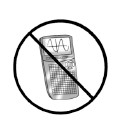asymptotes. Then, state the domain in parts (a) and (b) using interval notation and the domain in parts (c) and (d) using set notation. 1-90 HW eTool (Desmos). Homework Help ✎

1.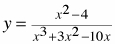2.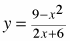3.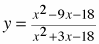4.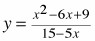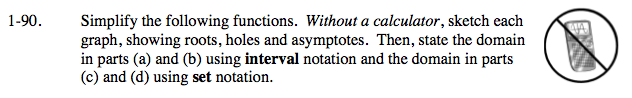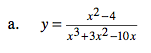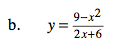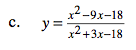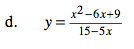$=\frac{(x+2)}{x(x+5)}$

Since (x − 2) cancelled out, x = 2 is the location of a hole.

Now simplify. And notice what was cancelled out.

The y-value of the hole can be found by evaluating the simplified function at x = 2.

$\frac{(2+2)}{(2)(2+5)}=\frac{4}{14}=\frac{2}{7}$

$\text{So the hole has a coordinate point of }\left (-2,\frac{2}{7} \right )$

x(x + 5) did not cancel out, so x = 0 and x = −5 are vertical asymptotes.

Now find the end behavior. You can long divide or use an approach statement: x → − ∞, y → 0 and as x → ∞, y → 0. There is a horizontal asymptote at y = 0.

Last but not least, investigate what is happening in the middle, by exploring the holes and vertical asymptotes. Use the simplified equation:

x → −5, y → −∞

x → 0+, y → +∞

x → −5+, y → +∞

$x\rightarrow 2^{-},\ y\rightarrow \frac{2}{7}$

x → 0, y → −∞

$x\rightarrow 2^{+},\ y\rightarrow \frac{2}{7}$

Use the eTool below to view the steps for graphing the first equation.
Click the link at right for the full version of the eTool: Calc 1-90 HW eTool